# 100+ Challenging math riddles to keep your mind sharp#### Rooster Eggs and Bananas - Math Riddle

###### 106.Math Riddles
`Can you solve this Viral Rooster Eggs and Bananas Equation Puzzle?`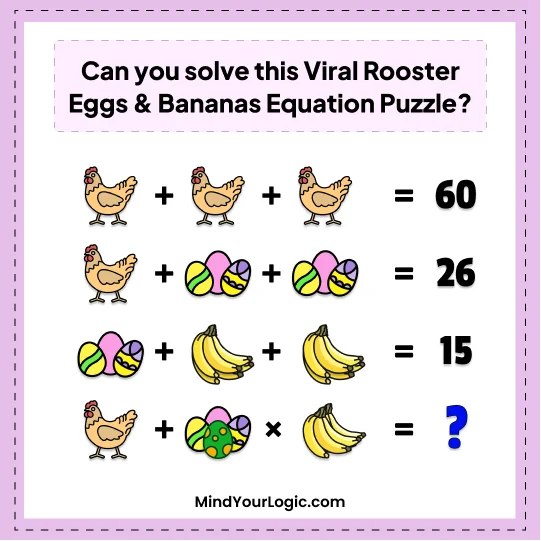• A.36
• B.40
• C.35
• D.50
A.36
```Explanation :

36
3 eggs = 3, one egg = 1 banana = 1.
so 1 chicken + 4 egg x 4 banana
= 20 + (4x4) = 20 + 16 = 36```

#### A fireman Jacob - Math Riddle

###### 107.Math Riddles
```A fireman Jacob stood at the center of a ladder and was spraying water on a burning house.
He climbed up 6 rungs before the heat of the flames forced him to come down 10 rungs.
Some minutes later, Jacob climb 18 rungs to the very top of the ladder.
How many rungs did the ladder have?```
• A.29
• B.30
• C.70
• D.40
A.29
```Explanation :

Jacob standing on the middle rung.He goes up 6 rungs so he is now at M+6 and when he goes down 10 rungs so he is at M-4.
Jacob goes up 18 rungs so he is now at M+14 = T
14 rungs from the top, 14 rungs from the bottom, and the middle rung.
14+14+1=29```

#### Picture equation - Math Riddle

###### 108.Math Riddles
`Can you solve the below picture equation brain teaser?`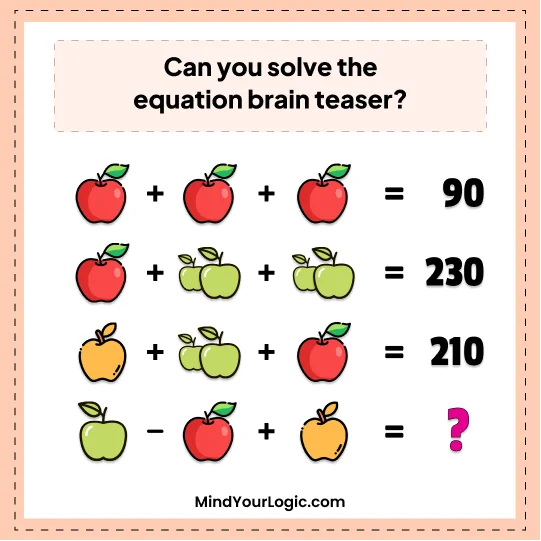• A.70
• B.120
• C.200
• D.100
D.100
```Explanation :

100
30+30+30=90
30+100+100=230
80+100+30=210
50-30+80= 100```

#### Fill the boxes - Math Riddle

###### 109.Math Riddles
```Fill the boxes using (1, 3, 5, 7, 9, 11, 13, 15)
You can also repeat the numbers```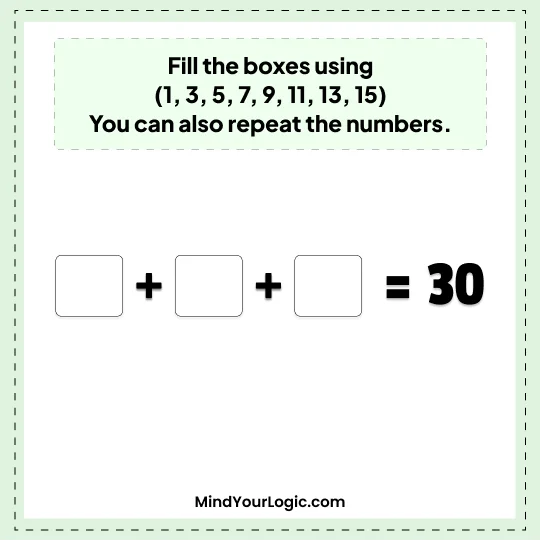`30`
```Explanation :

There can be multiple solutions to this problem.
Listing three of them
A) 11+13+6(rotating 9) = 30
B) 11+13+3! = 30
c) 11.5+5.5+13 = 30```

#### Jennifer decides to invest in Ethereum - Math Riddle

###### 110.Math Riddles
`Jennifer decides to invest in Ethereum. He pays \$12000 for it. After one day, the Ethereum value has increased to \$13000 and so Jennifer decides to sell it. But the next day she regrets her decision and she buys it again. Unfortunately, at a higher price and has to pay \$14000 to get it back, so she loses \$1000. The very next day, he sells the Ethereum for \$15000.`
`\$2,000 `
```Explanation :

\$2000
at first she bought it for 12000 and sold it 13000
Profit from 1st transaction = \$1000 (13000-12000)
next day she bought it for 14000 and sold it for 15000
Profit from 2nd transaction = \$1000 (15000-14000)
So the overall profit will be = (1st + 2nd )(1000+ 1000 )\$2000```

#### Who is not playing any game - Math riddle

###### 111.Math Riddles
```Who is not playing any game - Math riddle
A Group of 90 students is there in a sports group. "Football is played by 30 students. Volleyball is played by 40 students. Basketball is played by 25 students.
15 students play both Football and Volleyball.
10 students play both Football and Basketball.
8 students play both Volleyball and Basketball.
5 students are playing all the 3 games.
Can you find how many students are not playing any of the game ?```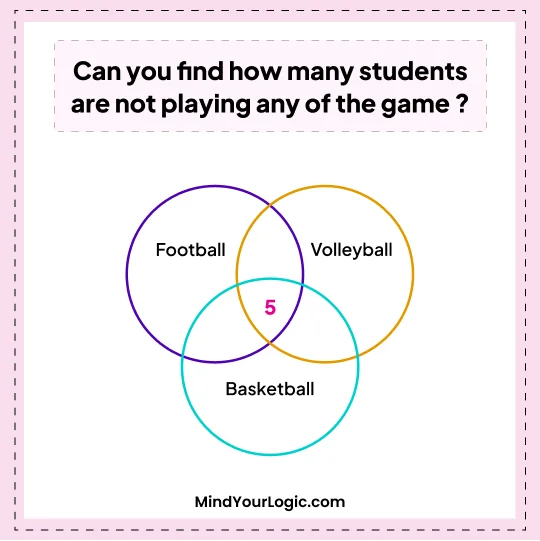• A.7
• B.3
• C.10
• D.5
D.5
```Explanation :

A total of 90 students are in the sports group.

30 students play football.
40 students play Volleyball.

15 students play both football and volleyball.
10 students play both football and basketball.
8 students play both volleyball and basketball.

5 students play all the three games.

Now, we will subtract the number of students playing each game from the total number of students in the group to find the number of students who are not playing any of the games.

First, let's find the number of students who are playing at least one game.

30 (students playing football) + 40 (students playing volleyball) + 25 (students playing basketball) - 15 (students playing both football and volleyball) - 10 (students playing both football and basketball) - 8 (students playing both volleyball and basketball) + 5 (students playing all three games) = 63

So, 63 students are playing at least one game.

Therefore, 90 (total number of students in the sports group) - 63 (students playing at least one game) = 27 students are not playing any of the games.```

#### Vehicle make scale balance - Math Riddle

###### 112.Math Riddles
`Which vehicle is needed to balance the scale?`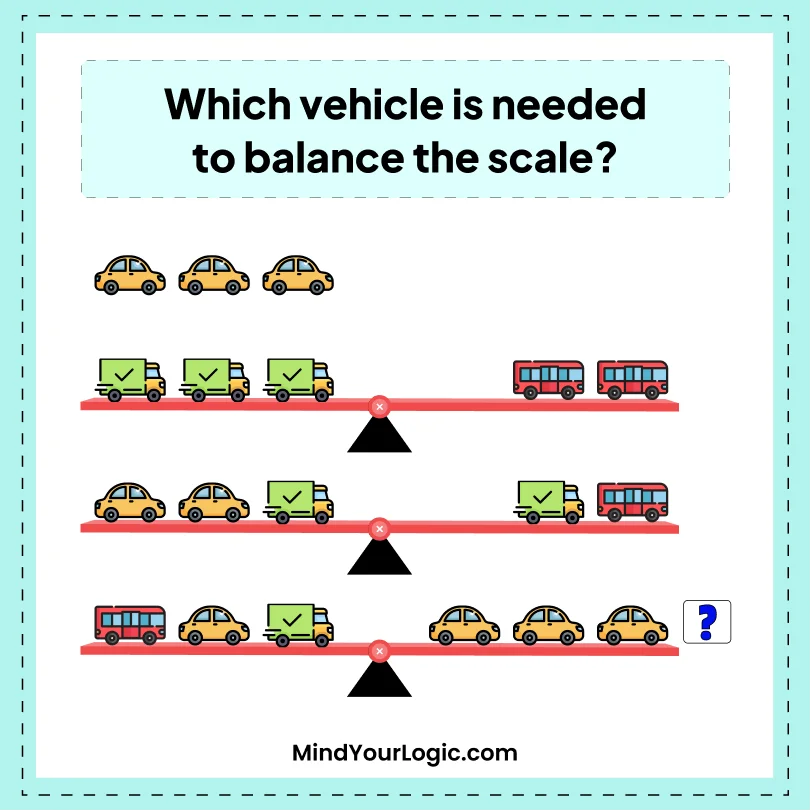• A.Carriage
• B.Bus
• C.Car
• D.none of them
A.Carriage
```Explanation :

Let us assume
Carriage = 2, the Car = 3 and the Bus = 6
6+3+2= 3+3+3
If we remove carriage it will be balancedCarriage = 2, the Car = 3 and the Bus = 6
6+3+2= 3+3+3
If we remove carriage it will be balanced```

#### Algebraic equation - Math Riddle

###### 113.Math Riddles
`Can you solve the Algebraic equation?`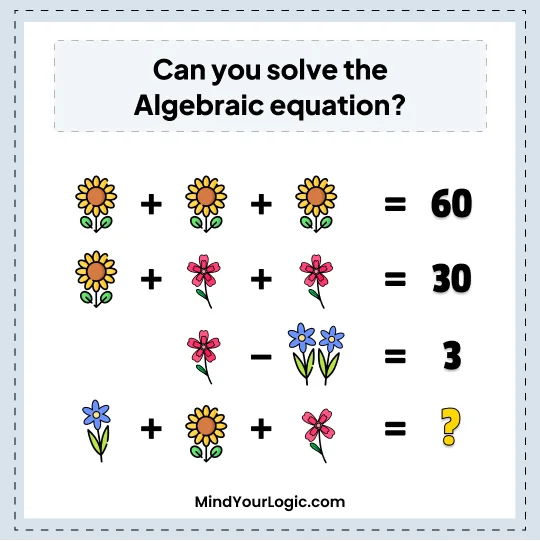• A.50
• B.30
• C.45
• D.25
D.25
```Explanation :

First Equation
1Red Flower + 1Red Flower + 1Red Flower = 60
Hence, 1Red Flower = 20
Second Equation
Red Flower + 5Blue Petals + 5Blue Petals = 30
20 + 10Blue Petals = 30
10Blue Petals = 10
Hence 1Blue Petals = 1
Third Equation
5Blue Petals + 2Yellow Flower = 3
5 + 2Yellow Flower = 3
2Yellow Flower = 2
Hence, 1Yellow Flower = 1
Required
Yellow Flower + Red Flower + 4Blue Petals = ?
Hence, 1 + 20 + 4 = 25```

#### Two soap of equal weights - Math Riddle

###### 114.Math Riddles
`We have two soap of equal weights & a 3/4 pound weight. However, after using 1/4 of soap we place all 3 objects on balance as on one side of balance we place one soap (unused) & on another side, We placed used soap (i.e 3/4th of soap) & 3/4 pound weight. What is the soap weight?`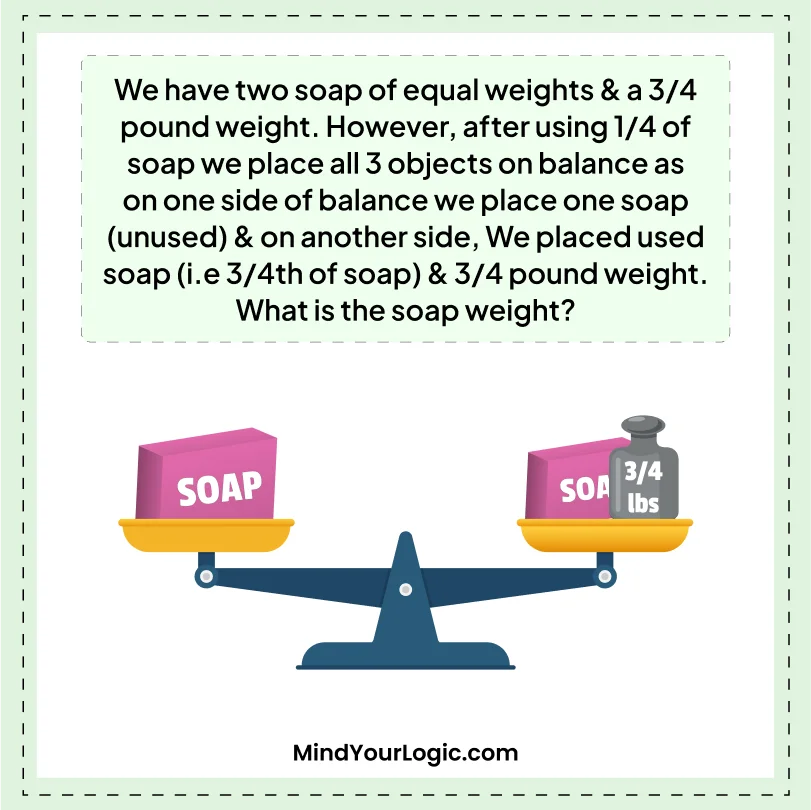`3 lbs.`
```Explanation :

If the balance was equal when one soap was on one side and the other soap and a 3/4 pound weight were on the other side, we can use this information to find the weight of the soap.

Let's call the weight of the soap "x". Then, when one soap was on one side, the weight on the other side was (3/4) + (3/4) * x. Since the balance was equal, the weight of the two sides must be equal, so we can set them equal to each other and solve for x:

x = (3/4) + (3/4) * x

Solving for x, we get:

x = 3/4 + 3/4x

Subtracting 3/4x from both sides:

1/4x = 3/4

Dividing both sides by 1/4:

x = 3

So the weight of each soap is 3 pounds.```

#### Four digit number based on three clues - Math Riddle

###### 115.Math Riddles
```Can you find a four digit number based on three clues below?
Clue 1: Its first digit is of no use.
Clue 2: Its second and fourth digit is the mirror image of each other.
Clue 3: The third digit is half the second.```
`848`
```Explanation :
Clue 1: Its first digit is of no use.
The digit '0' at the start of the number is of no use.

Clue 2: Its second and fourth digit is the mirror image of each other.
The number '8' is the only digit that is the mirror image of itself.

Clue 3: The third digit is half the second.
4 is half of 8```

#### The height of red bar - Math Riddle

###### 116.Math Riddles
`Can you determine the height of red bar with the help of information provided in the picture below?`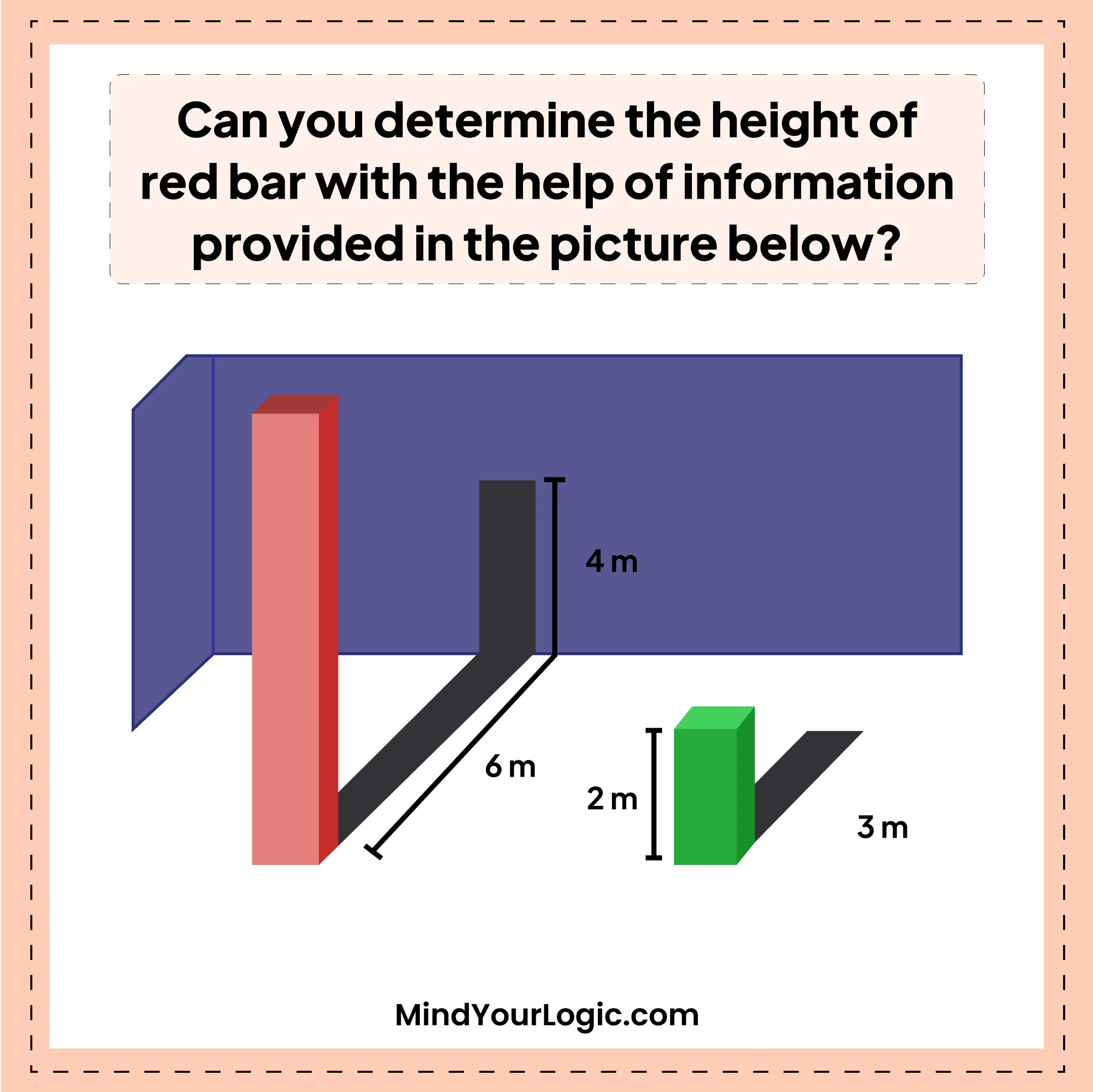• A.8
• B.6
• C.10
• D.15
A.8
```Explanation :

8m
From green bar, we can deduce that
the height of vertical bar = 2/3 of uninterrupted shadow length of the bar.
Therefore shadow of 6m of red bar indicates the height of 4m.
The remaining red bar casts a direct 1:1 vertical projection on the wall.
Therefore total length = 4m + 4m = 8m.```

#### Identify the logic - Math Riddle

###### 117.Math Riddles
```Can you identify the logic behind the equation below and solve the puzzle by completing the equation?
12 + 13 + 7 = 1, 15 + 16 + 11= 2, 17 + 18 + 16 = ?```
• A.5
• B.4
• C.7
• D.9
B.4
```Explanation :

Logic Used: Sum the numbers and then subtract the two digits to get the desired answer.
12 + 13 + 7 => 32 => 3-2 => 1
15 + 16 + 11 =>42 => 4-2 => 2
17 + 18 + 16 = 51 => 5-1 => 4```

#### Replace each alphabet with the number - Math Riddle

###### 118.Math Riddles
```Can you replace each letter with the number (1 - 9) to make all equation correct ?

AB - C = DE / F = GH * I ```
`39`
```Explanation :

45-6 = 78 / 2 = 39 * 1

A = 4
B = 5
C = 6
D = 7
E = 8
F = 2
G = 3
H = 9
I = 1

So below equation can be solved as:
AB - C = DE / F = GH * I
45 - 6 = 78/2 = 39 * 1
39 = 39 = 39.```

#### Considering below equationr - Math Riddle

###### 119.Math Riddles
```Considering below equation holds
V + II - VI = 10 ,
IV - X + VIII = 20 ,
IV - VII + VI = 30
Then, I + II + III = ?```
• A.50
• B.80
• C.70
• D.60
D.60
```Explanation :

60
V + II   VI = 10
= I = 10
IV   X + VIII = 20
= II = 20
IV   VII + VI = 30
= III = 30
I + II + III = 10 + 20 + 30```